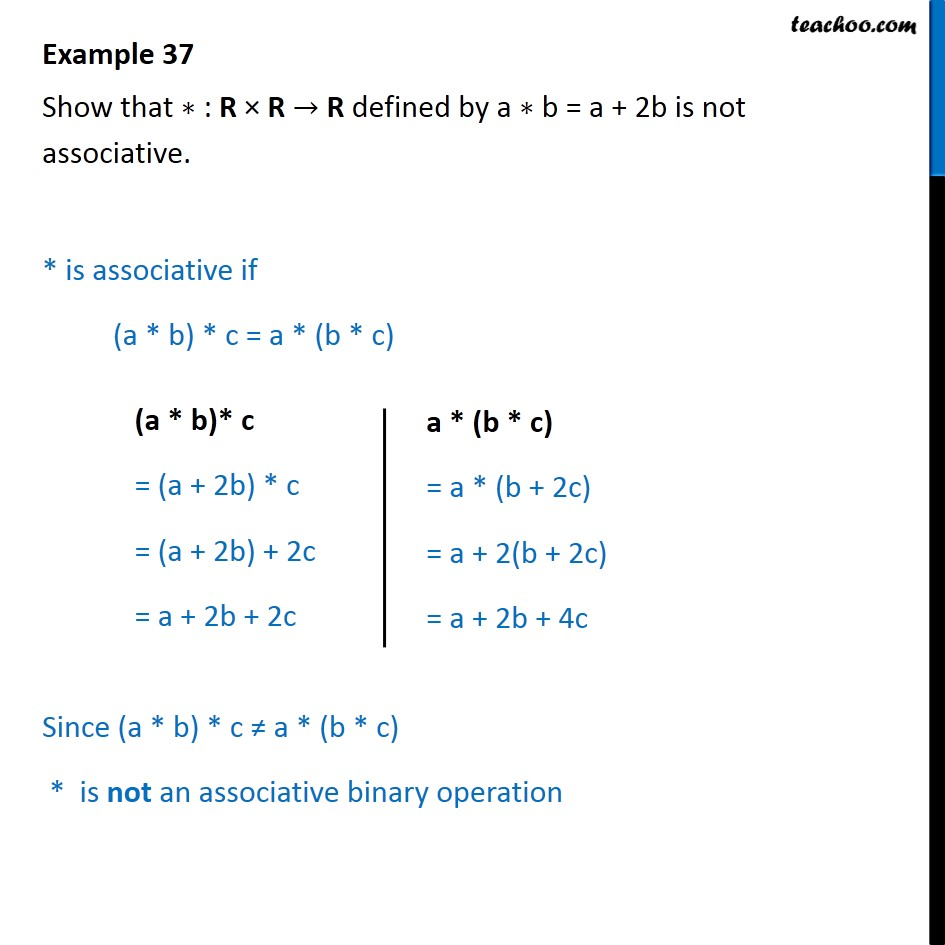1. Chapter 1 Class 12 Relation and Functions (Term 1)
2. Serial order wise
3. Examples

Transcript

Example 37 Show that ∗ : R × R → R defined by a ∗ b = a + 2b is not associative. * is associative if (a * b) * c = a * (b * c) Since (a * b) * c ≠ a * (b * c) * is not an associative binary operation

Examples

Chapter 1 Class 12 Relation and Functions (Term 1)
Serial order wise